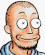# golang: types such as []uint32 and cgo (2012)

in tag golang

There is official documentation on the Go C language interface (or `cgo` in golang terminology), but the things it covers are relatively simple. I have used cgo recently in a real-world project and I want to share my experiences in this short article, that is, how to use types properly (avoiding the `void*` equivalent `unsafe.Pointer`) and how to deal with Go’s data structures such as slices.

## A simple example

To make sure we’re on the same page, let’s consider this simple example:

``````package main
import "fmt"
func main() {
list := []int{23, 42, 17}
for idx, val := range list {
fmt.Printf("index %d: value %d\n", idx, val)
}
}
``````

The output of that program is:

```index 0: value 23
index 1: value 42
index 2: value 17
```

## Multiplying these numbers

Let’s assume that we want to multiply all these numbers by 2. In Go, that’s pretty simple:

``````package main
import "fmt"

func multiply(input []int) []int {
// Create an output list with the same size
output := make([]int, len(input))
for idx, val := range input {
output[idx] = val * 2
}
return output
}

func main() {
list := []int{23, 42, 17}
list = multiply(list)
for idx, val := range list {
fmt.Printf("index %d: value %d\n", idx, val)
}
}
``````

Now let’s see how we would do that in C with cgo. Note that we switch to using `uint32` instead of `int` because that makes the point I’m trying to make easier to convey.

``````package main
import "fmt"

/*
// Note the -std=gnu99. Using -std=c99 will not work.
#cgo CFLAGS: -std=gnu99
#include <stdint.h>

void cMultiply(int len, uint32_t *input, uint32_t *output) {
for (int i = 0; i < len; i++) {
output[i] = input[i] * 2;
}
}
*/
import "C"

func multiply(input []uint32) []uint32 {
output := make([]uint32, len(input))
C.cMultiply(C.int(len(input)),
(*C.uint32_t)(&input),
(*C.uint32_t)(&output))
return output
}

func main() {
list := []uint32{23, 42, 17}
list = multiply(list)
for idx, val := range list {
fmt.Printf("index %d: value %d\n", idx, val)
}
}
``````

As you can see, we need to convert the Go types into C types, which can be done by simply type-casting them. Also, we need to manually implement the array calling convention which is normally done by the C compiler: We pass a pointer to the first element.

We have also avoided passing the slice directly to the C code and instead passed the length plus a pointer to the contents. This is a simple way to avoid having to use the internal Go `SliceHeader` data type.

If you are using C code to speed up some critical routines, you might want to throw in a `-O3` in the `#cgo CFLAGS` pragma.

It is noteworthy that you should avoid calling a lot of cgo-functions, since the function call overhead is much higher than the normal go function call overhead.